f
Neutrium

# Fluid Flow

Cv versus Orifice SizeThe pressure drop or flow rate through a valve or orifice plate is typically calculated using the a flow coefficient, Cv or orifice diameter. This article demonstrates how to convert between these two parameters when performing functions such as selecting a valve with an equivalent pressure drop to a given orifice plate.

Choked Flow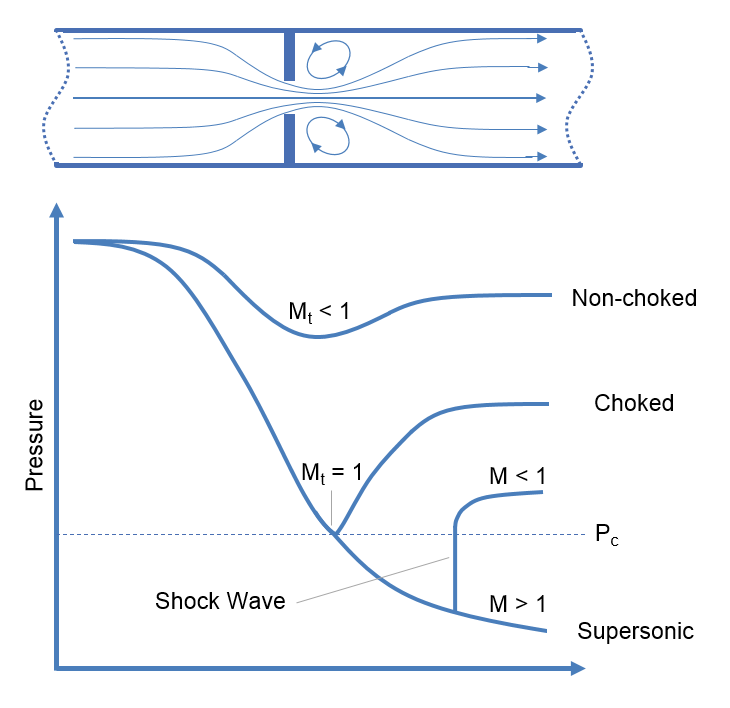Choked flow is a phenomenon that limits the mass flow rate of a compressible fluid flowing through nozzles, orifices and sudden expansions. Generally speaking it is the mass flux after which a further reduction in downstream pressure will not result in an increase in mass flow rate.

Erosion Velocity for Gas-Liquid Multiphase Flow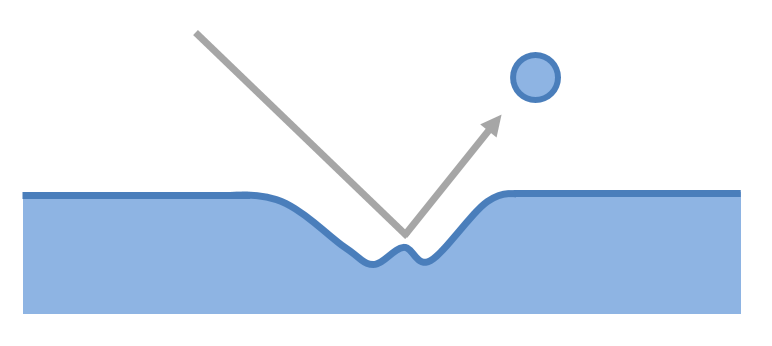The flow of a gas-liquid multiphase system may cause erosion if velocities are high. This article presents an empirical relationship for estimating whether erosion will occur in a system at a certain velocity.

Joukowsky Equation

The Joukowsky equation is a method of determining the surge pressures that will be experienced in a fluid piping system. When a fluid in motion is forced to either stop or change direction suddenly a pressure wave will be generated and propagated through the fluid. This pressure wave is commonly referred to as fluid hammer (also known as water hammer, surge or hydraulic shock) and typically occurs in piping systems when a valve is suddenly closed, isolating the line. The resultant surge pressures are complex to characterise but for simple systems they may be calculated using the Joukowsky equation.

Flow in Open Channels and Partially Filled Pipes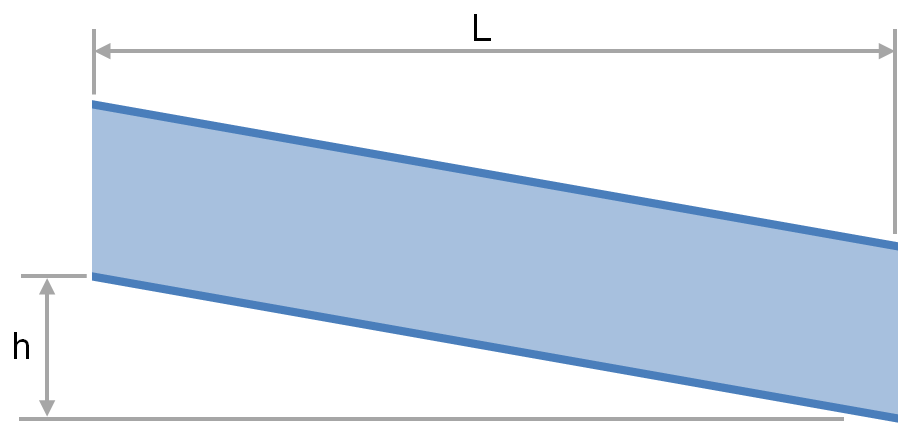The transport of fluid under gravity is often achieved using partially filled pipes, channels, flumes, ditches and streams. To determine the slope and elevation change required or the flow rate that is achievable one must be able to calculate the head loss and friction factor. This article provides relationships for the calculation of head loss and friction factor for fluids flowing via these conduits.

Comparing Volumetric Flow Rates for Equivalent Pressure Loss

Standard volumetric flow rates of a fluid are often used to describe the capacity of a vent or pressure relief device. To determine how this capacity compares for another fluid under different pressure and temperature conditions a conversion must be made on the basis of equivalent pressure loss. This article describes the method for calculating the volumetric flow rate of a gas which will give the equivalent pressure drop to another gas through a fixed restriction such as a vent.

Torricelli's Law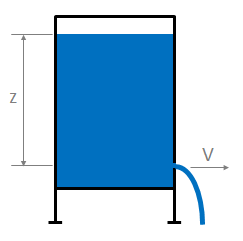This article presents Torricelli’s law, a simplified method of estimating the velocity of fluid passing through an open orifice under static pressure.

Bernoulli's Equation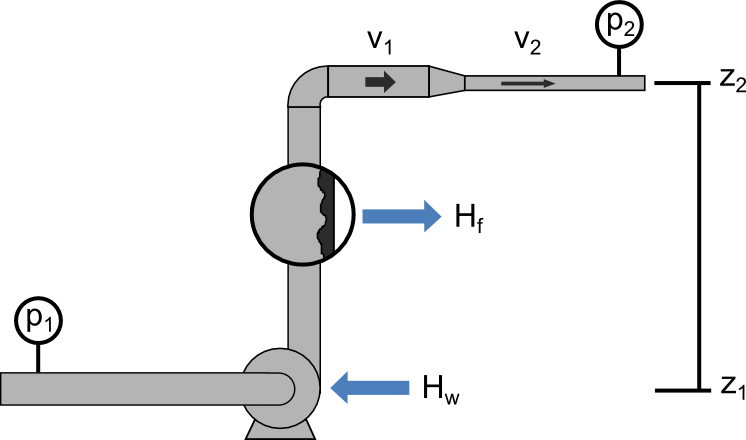Bernoulli’s Principle is an important observation in fluid dynamics which states that for an inviscid flow, an increase in the velocity of the fluid results in a simultaneous decrease in pressure or a decrease in the fluid’s potential energy. This principle is often represented mathematically in the many forms of Bernoulli’s equation. This article presents some useful forms of Bernoulli’s Equations and their simplifying assumptions.

Pressure Loss in Hoses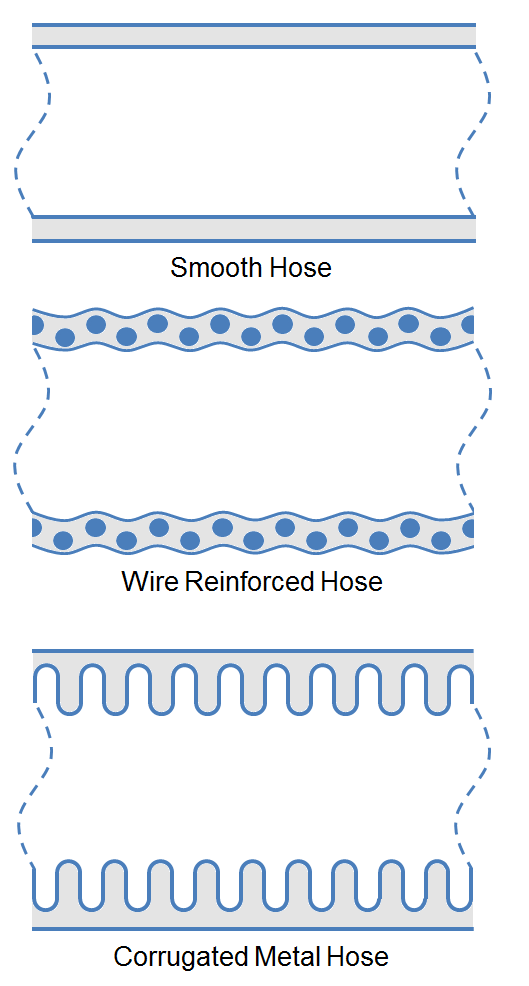The Pressure loss through a hose is often approximated using coarse heuristics, but utilization of more accurate correlations increase the efficiency of pump and piping designs. This article presents more accurate methods to estimate the pressure loss in various type of hoses using multiples of the pipe length. Methods of estimating pressure loss caused by couplings, curves and coiled hose are also detailed.

Calculating Interface Volumes for Multi-product Pipelines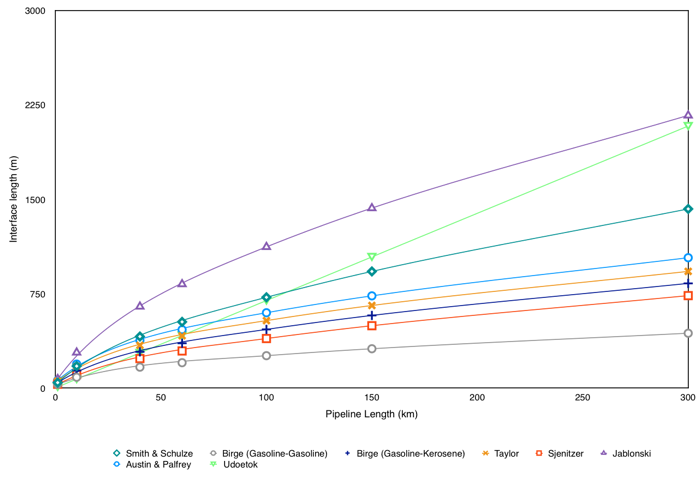Due to their large capital expense, pipelines are often utilized for the transfer of multiple products. During operation of these multi-product pipelines, the interface between two adjacent products extends (referred to as interface mixing), resulting in the contamination of each product. This interface is typically sent to slops collection for reprocessing or disposal at additional cost to the operator. Therefore the economics of a pipeline can often be improved through a study of product interfaces under various operational conditions to aide in the minimization of interface mixing. This article presents several empirical methods by which interface mixing can be quantified.